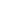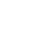## Free, outstanding support from WebAssist and your colleagues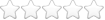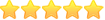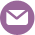# Specific pricing based on quantity entered

Thread began 4/23/2009 1:00 pm by Lee Reynolds | Last modified 5/01/2009 10:39 am by Lee Reynolds | 3895 views | 9 replies |

##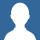4/23/2009 1:00 pm  |  #1 Lee Reynolds

### Specific pricing based on quantity entered

Perhaps I'm not looking in the right place, so any help would be appreciated. I have a cart that needs to calculate a specific price based on a quantity entered off of a sliding scale for a class. A quantity of 1 is charged \$2000, the second person on the order gets in for \$750 (so total of 2750), then 3rd or more get in for 500 each (so 4 would be 2000+750+500+500). Any easy way to set this?

##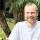4/23/2009 2:39 pm  |  #2 Ray BorduinWebAssist

Yes, you can do this. How I would do it would depend on my application and how many items I sell and how much variation in this formula I would need to support. For the most flexability, you would create two additional columns in your cart named "SecondDiscount" and "MultipleDiscount".

Then you can go to the calculations tab and update the totalprice calculation from:

[Price] * [Quantity]

to:

([Price] * [Quantity]) - (([Quantity]>1)?(([Quantity]-1)*[SecondDiscount]):0) - (([Quantity>2])?(([Quantity]-2)*[MultipleDiscount]):0)

And then your values when you entered into the cart would be:

Price: 2000
SecondDiscount: 1250
MultipleDiscount: 250

Did this help? Tips are appreciated...##4/30/2009 8:39 pm  |  #3 Lee Reynolds

Ray -- thanks for the message, but I'm confused a bit :)

I can get the calculation working in PHP by doing this:

<?php
// set vars
\$Quantity = \$_POST['quantity']; // selected on previous page
\$Price = \$recordset['price'] // variable and set in DB, pulled in recordset
// calculate!
if (\$Quantity == 1)
\$Price = \$Price;
elseif (\$Quantity == 2)
\$Price = \$Price+750;
else //(\$Quantity >= 3)
\$Price = \$Price+750+((\$Quantity-2)*500);
?>

so...

I think the cart calculation should be something like this:

(([Quantity]=1)?([Price]) OR
(([Quantity]=2)?([Price])+750 OR
(([Quantity]>2)?([Price]+750)*(([Quantity]-2)*500))

I'd prefer for this not to appear as a discount line then calculate -- that will confuse the heck out of folks. This is similar to buy one at regular price, get a second for \$10 and as many others as you want (3rd or more) for \$5 each (insert numbers as needed).

Thoughts? Thanks again.

##5/01/2009 4:59 am  |  #4 Ray BorduinWebAssist

That is exactly what my calculation does.

Say that you had a cart with the columns:
Quantity: 2
Price: 2000
SecondDiscount: 1250
MultipleDiscount: 250

and you use the formula I gave of:
([Price] * [Quantity]) - (([Quantity]>1)?(([Quantity]-1)*[SecondDiscount]):0) - (([Quantity>0])?(([Quantity]-2)*[MultipleDiscount]):0)

I'll replace the numbers:
(2000 * 2) - ((2>1)?((2-1)*1250):0) - ((2>2)?((2-2)*250):0)

Then I'll combine a few:

(4000) - ((2>1)?(1250):0) - ((2>2)?(0):0)

the syntax ((x)?y:z) will return y if x is true and z if it is false, so above, ((2>1)?(1250):0) will return 1250 because it is true that 2>1. Using that I can further simplify the result to:

4000-1250 or 2750

Which is the expected result given your parameters.

What I did is I combined tha three line OR statement that you gave:

(([Quantity]=1)?([Price]) OR
(([Quantity]=2)?([Price])+750 OR
(([Quantity]>2)?([Price]+750)*(([Quantity]-2)*500))

and I used algebra and php to combine it into a single calculation.

Did this help? Tips are appreciated...##5/01/2009 6:28 am  |  #5 Lee Reynolds

OK. I see how you're getting there.

Shouldn't the second calculation be [Quantity>2] instead of [Quantity>0]? If you have only one participant, 1>0 and will trigger the third part. In your sample, 2 is greater than zero and succeeds in that 2-2 = 0 nullifying that part but only works of quantity = 2.

There is also an add-to-cart element that does not use this calculation. Is best practice to add the two columns and add a separate calculation just for this part? (the separate part is that participants can make a deposit of \$500 per person to hold space -- the rest is payable later). I can show you the sample page.

Thanks for your help again.

##5/01/2009 6:30 am  |  #6 Ray BorduinWebAssist

If it doesn't use the calculation, just set both of the columns for SecondDiscount and MultipleDiscount to zero.

Yes, it should be a 2... looks like you must be following the logic now that you are correcting it ;)

Did this help? Tips are appreciated...##5/01/2009 6:38 am  |  #7 Lee Reynolds

I haven't had algebra since the mid 1980s and my brain hurts. I'll follow up with the result. I think this may occur more than once.

##5/01/2009 8:06 am  |  #8 Lee Reynolds

Ray --

It works! Thanks again! I need to work through all the events to make sure this is water tight. :)

##5/01/2009 8:38 am  |  #9 Ray BorduinWebAssist

you are missing a "(" after the ? in both... it should be:

([Price] * [Quantity]) - (([Quantity]>1)?(([Quantity]-1)*[SecondDiscount]):0) - (([Quantity]>2)?(([Quantity]-2)*[MultipleDiscount]):0)

Did this help? Tips are appreciated...##5/01/2009 10:39 am  |  #10 Lee Reynolds

Ray --

Just a followup as promised. One thing I didn't account for is that the base price of the event changes for each course. So rather than a discount, it has to be a surcharge based off of the base price:

2000+750+500+500=3750;
4000+750+500+500=4750

The base price is found in a database column for each event (class/seminar).

From all of your help and leveraging your logic, this formula works even of the base price for the event changes:

([Price]) + (([Quantity]>1)?([Price]-([Price]-[SecondDiscount])):0) + (([Quantity]>2)?(([Quantity]-2)*([Price]-([Price]-[MultipleDiscount]))):0)

Thank you so much for your help -- have a great weekend!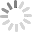## Build websites with a little help from your friends

Your friends over here at WebAssist! These Dreamweaver extensions will assist you in building unlimited, custom websites.

## Build websites from already-built web applications

These out-of-the-box solutions provide you proven, tested applications that can be up and running now.  Build a store, a gallery, or a web-based email solution.

## Everything else!

Close Window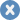### Rate your experience or provide feedback on this page

Account or customer service questions?
Please user our contact form.

Need technical support?
Please visit support to ask a question

ContentLayoutEase of use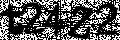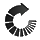We do not respond to comments submitted from this page directly, but we do read and analyze any feedback and will use it to help make your experience better in the future.

Close Window## We were unable to retrieve the attached file

Close Window### Attach and remove files

• Text Formatting
• Insert Element
• Block Formatting
• Cancel
Close Window#### Enter the URL you would like to link to in your post

Close Window#### This is how you use right click RTF editing

Enable right click RTF editing option allows you to add html markup into your tutorial such as images, bulleted lists, files and more...-- click to close --